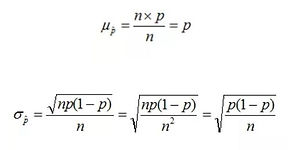## Chapter 6 - Day 6 - Lesson 6.4

##### Learning Targets
• Calculate the mean and standard deviation of the sampling distribution of a sample proportion and interpret the standard deviation.

• Determine if the sampling distribution of the sample proportion is approximately normal.

• If appropriate, use a normal distribution to calculate probabilities involving the sample proportion.

##### Activity: Is this a fair coin? Part 2

Today we used our coin flip data from lesson 6.3.  Students turned the number of heads they flipped out of 30 (sample counts) into a proportion (sample proportions).  Once they had converted into the proportions, they wrote the outcomes on a sticker and added it to the class dotplot.  It was really nice to have the lesson 6.3 sticker dotplot that we had made posted so students could reference it easily.  We posted the dotplots next to each other and had the students compare and contrast.  One mistake we made was not using a consistent scale on the axes.  As you can see in our dotplots, the scale on the a sample proportions dotplot is a little wider.  Because of this, many students thought the sample proportions were more variable than the sample counts.  We had to then draw their attention to the height of each bar for them to realize the distributions are actually the same except for the labels on the axes are different.

After comparing the distributions, students brainstormed about how to calculate the new mean and standard deviation.  Because they had just divided their sample counts by 30 repeatedly to make a proportion, most groups divided the mean and standard deviation as well.  We challenged students to try to write a formula for each using n and p.This was a great connection for them to understand how we got the formulas for the mean and standard deviation of a sampling distribution of proportions.  Also, if students forget the reduced formulas, they can always work their way there using the sample counts.  For example, many students would calculate the standard deviation for the counts and then would divide it by n.  This shows a conceptual understanding for what they are calculating. Don’t forget to fill out the formula review sheet!

##### Teaching Tip:

Make sure to keep your dotplots each day for each class! Make a new dotplot for each hour and post them in the room.  This was a helpful reminded throughout the chapter to students of what they had learned.